SLVSC81C September   2013  – February 2016

PRODUCTION DATA.

1. Features
2. Applications
3. Description
4. Revision History
5. Pin Configuration and Functions
6. Specifications
7. Detailed Description
1. 7.1 Overview
2. 7.2 Functional Block Diagram
3. 7.3 Feature Description
4. 7.4 Device Functional Modes
8. Application and Implementation
1. 8.1 Application Information
2. 8.2 Typical Application
9. Power Supply Recommendations
10. 10Layout
11. 11Device and Documentation Support
12. 12Mechanical, Packaging, and Orderable Information

• DBV|5

## 8 Application and Implementation

NOTE

Information in the following applications sections is not part of the TI component specification, and TI does not warrant its accuracy or completeness. TI’s customers are responsible for determining suitability of components for their purposes. Customers should validate and test their design implementation to confirm system functionality.

### 8.1 Application Information

The TPS560200 is used as a step-down converter which converts a voltage of 4.5 V to 17 V to a lower voltage. WEBENCH® software is available to aid in the design and analysis of circuits.

### 8.2 Typical Application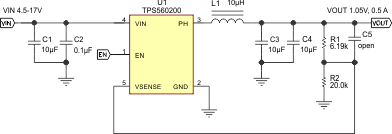Figure 7. Typical Application Schematic

#### 8.2.1 Design Requirements

To begin the design process, the user must know a few application parameters:

### Table 1. Design Parameters

PARAMETER VALUES
Input voltage range 4.5 V to 17 V
Output voltage 1.05 V
Output current 500 mA
Output voltage ripple 10 mV/pp

#### 8.2.2.1 Output Voltage Resistors Selection

The output voltage is set with a resistor divider from the output node to the VFB pin. TI recommends using 1% tolerance or better divider resistors. Start by using Equation 2 to calculate VOUT.

To improve efficiency at light loads, consider using larger value resistors, high resistance is more susceptible to noise, and the voltage errors from the VSENSE input current are more noticeable.

Equation 2.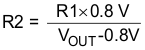#### 8.2.2.2 Output Filter Selection

The output filter used with the TPS560200 is an LC circuit. This LC filter has double pole at:

Equation 3.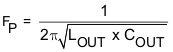At low frequencies, the overall loop gain is set by the output set-point resistor divider network and the internal gain of the TPS560200. The low frequency phase is 180 degrees. At the output filter pole frequency, the gain rolls off at a –40 dB per decade rate and the phase drops rapidly. D-CAP2 introduces a high frequency zero that reduces the gain roll off to –20 dB per decade and increases the phase to 90 degrees one decade above the zero frequency. The inductor and capacitor selected for the output filter must be selected so that the double pole of Equation 3 is located below the high frequency zero but close enough that the phase boost provided by the high frequency zero provides adequate phase margin for a stable circuit. To meet this requirement use the values recommended in Table 2.

### Table 2. Recommended Component Values

Output Voltage
(V)
R1
(kΩ)
R2
(kΩ)
C5
(pF)
L1
(µH)
C3 + C4
(µF)
MIN TYP MAX
1.0 4.99 20.0 10 10 + 10
1.05 6.19 20.0 10 10 + 10
1.2 10.0 20.0 10 10 + 10
1.5 17.4 20.0 10 10 + 10
1.8 24.9 20.0 optional 10 10 + 10
2.5 42.2 20.0 optional 10 10 + 10
3.3 61.9 20.0 optional 10 10 + 10
5.0 105 20.0 optional 10 10 + 10

Because the DC gain is dependent on the output voltage, the required inductor value increases as the output voltage increases. Additional phase boost can be achieved by adding a feed-forward capacitor (C5) in parallel with R1. The feed-forward capacitor is most effective for output voltages at or above 1.8 V.

The inductor peak-to-peak ripple current, peak current, and RMS current are calculated using Equation 4, Equation 5, and Equation 6. The inductor saturation current rating must be greater than the calculated peak current and the RMS or heating current rating must be greater than the calculated RMS current. Use 600 kHz for fSW.

Use 600 kHz for fSW. Make sure the chosen inductor is rated for the peak current of Equation 5 and the RMS current of Equation 6.

Equation 4.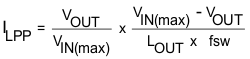Equation 5.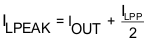Equation 6.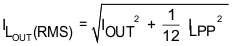For this design example, the calculated peak current is 0.582 A and the calculated RMS current is 0.502 A. The inductor used is a Würth 744777910 with a peak current rating of 2.6 A and an RMS current rating of 2 A.

The capacitor value and ESR determines the amount of output voltage ripple. The TPS560200 is intended for use with ceramic or other low-ESR capacitors. The recommended values are given in Table 2. Use Equation 7 to determine the required RMS current rating for the output capacitor.

Equation 7.For this design two MuRata GRM32DR61E106KA12L 10-µF output capacitors are used. The typical ESR is 2 mΩ each. The calculated RMS current is 0.047 A and each output capacitor is rated for 3 A.

#### 8.2.2.3 Input Capacitor Selection

The TPS560200 requires an input decoupling capacitor and a bulk capacitor is needed depending on the application. A ceramic capacitor over 10 μF is recommended for the decoupling capacitor. An additional 0.1-µF capacitor (C2) from pin 4 to ground is optional to provide additional high frequency filtering. The capacitor voltage rating must be greater than the maximum input voltage.

#### 8.2.3 Application Curves

VIN = 12 V, VOUT = 1.05 V, TA = 25°C (unless otherwise noted).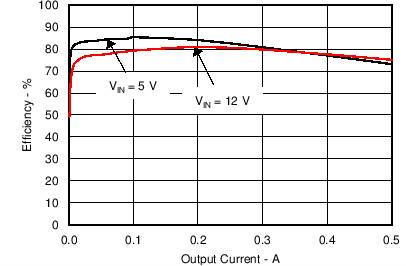Figure 8. EfficiencyFigure 10. Load Regulation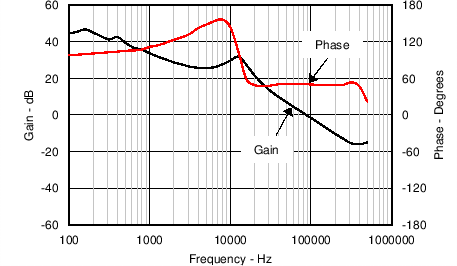Figure 12. Loop Response, IOUT = 0.25 A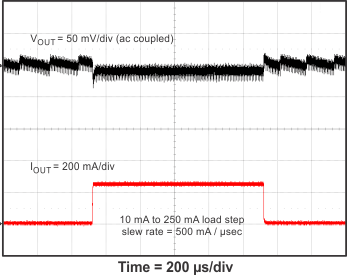Figure 14. Transient Response, 2% to 50% Load Step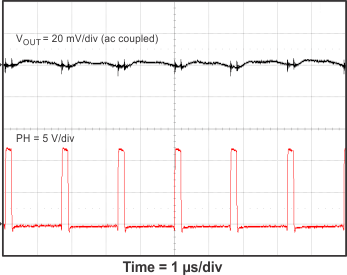Figure 16. Output Ripple, IOUT = 500 mA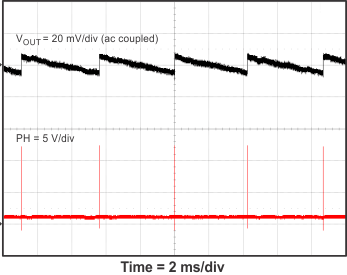Figure 18. Output Ripple, IOUT = 0 mAFigure 9. Light-Load Efficiency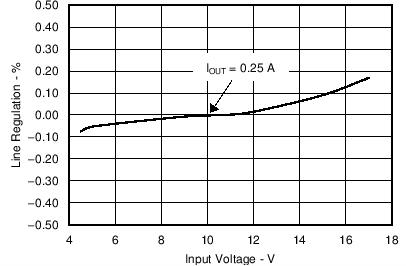Figure 11. Line Regulation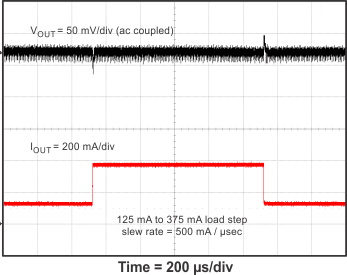Figure 13. Transient Response, 25% to 75% Load Step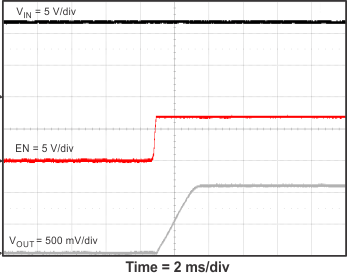Figure 15. Start-Up Relative to EN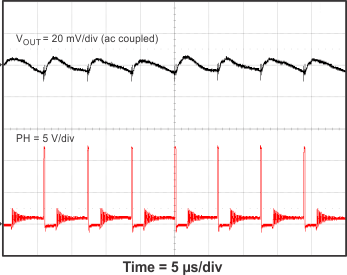Figure 17. Output Ripple, IOUT = 30 mA